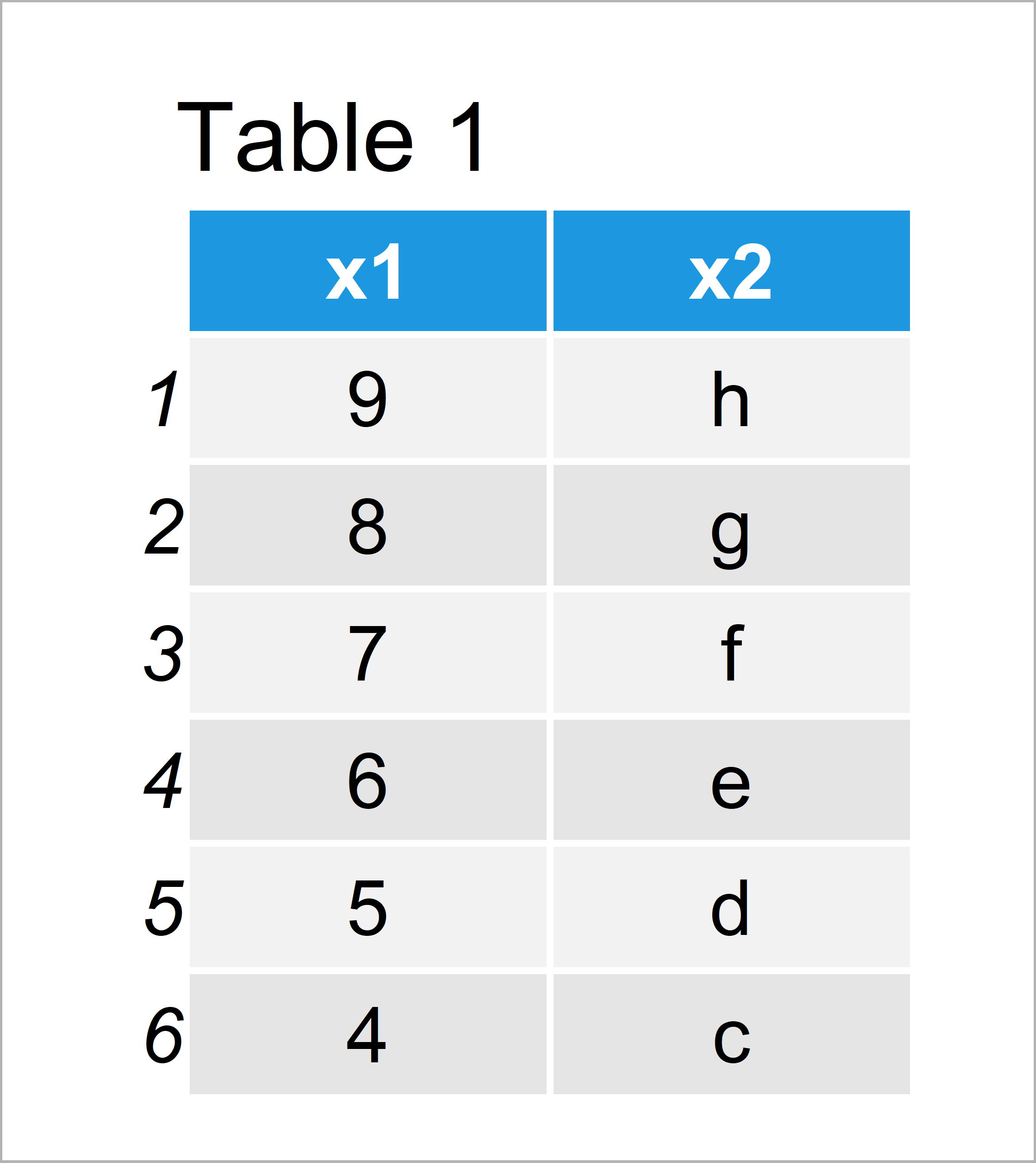# Add Multiple New Columns to data.table in R (Example)

On this page you’ll learn how to append several new data.table variables in the R programming language.

So let’s dig in.

## Example Data & Packages

We first need to install and load the data.table package, to be able to use the functions that are contained in the package:

```install.packages("data.table")      # Install & load data.table
library("data.table")```

We’ll use the following data.table as basement for this R programming tutorial:

```data <- data.table(x1 = 9:4,        # Create example data.table
x2 = letters[8:3])
data                                # Print example data.table```As you can see based on Table 1, our example data is a data.table constructed of six data points and four columns.

Next, we have to create two vectors that we can add as new columns to our data.table. The first vector looks like this…

```y1 <- 1:6                           # Create first new column
y1                                  # Print first new column
#  1 2 3 4 5 6```

…and the second vector is shown below:

```y2 <- LETTERS[1:6]                  # Create second new column
y2                                  # Print second new column
#  "A" "B" "C" "D" "E" "F"```

Let’s join all these data!

## Example: Add Multiple Columns to data.table Using `:=`

This example illustrates how to add several vectors as new variables to a data.table object in only one function call.

Have a look at the following line of R code:

`data[ , `:=` (y1 = y1, y2 = y2)]    # Add columns to data.table`

data # Print updated data.tableAfter executing the previous R programming syntax the data.table revealed in Table 2 has been created. As you can see, we have added the two vector objects to our input data.table.

## Video, Further Resources & Summary

If you need more explanations on the R programming codes of the present tutorial, you might watch the following video of my YouTube channel. I’m explaining the R programming codes of this post in the video:

Furthermore, you might want to read the other articles on Statistics Globe.

Summary: In this R tutorial you have learned how to combine multiple vectors and a data.table. Don’t hesitate to let me know in the comments section below, in case you have additional comments or questions.

Subscribe to the Statistics Globe Newsletter